# MP Board Class 10th Maths Solutions Chapter 13 Surface Areas and Volumes Ex 13.4

In this article, we will share MP Board Class 10th Maths Book Solutions Chapter 13 Surface Areas and Volumes Ex 13.4 Pdf, These solutions are solved subject experts from the latest edition books.

## MP Board Class 10th Maths Solutions Chapter 13 Surface Areas and Volumes Ex 13.4

Use π = $$\frac{22}{7}$$, unless stated otherwise.

Question 1.
A drinking glass is in the shape of a frustum of a cone of height 14 cm. The diameters of its two circular ends are 4 cm and 2 cm. Find the capacity of the glass.
Solution:
We have,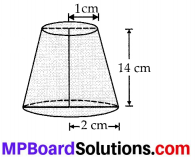d1 = 4 cm
∴ r1 = $$\frac{d_{1}}{2}$$ = 2 cm
and d2 = 2 cm
r2 = $$\frac{d_{2}}{2}$$ = 1 cm
and h = 14 cm
Volume of the glass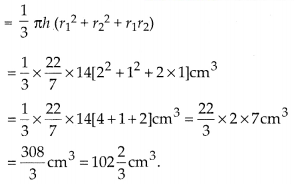Question 2.
The slant height of a frustum of a cone is 4 cm and the perimeters (circumference) of its circular ends are 18 cm and 6 cm. Find the curved surface area of the frustum.
Solution:
We have,
Slant height (l) = 4 cm
Circumference of one end = 2πr1 = 18 cm
and Circumference of other end = 2πr2 = 6 cm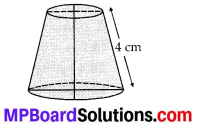⇒ πr1 = $$\frac{18}{2}$$ = 9 cm
and πr2 = $$\frac{6}{2}$$ = 3 cm
∴ Curved surface area of the frustum of the cone
= π(r1 + r2) l = (πr1 + πr2) l = (9 + 3 ) × 4 cm2
= 12 × 4 cm2 = 48 cm2.

Question 3.
A fez, the cap used by the Turks, is shaped like the frustum of a cone (see figure). If its radius on the open side is 10 cm, radius at the upper base is 4 cm and its slant height is 15 cm, find the area of material used for making it.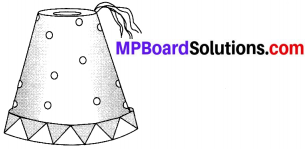Solution:
Here, the radius of the open side (r1) = 10 cm
The radius of the upper base (r2) = 4 cm
Slant height (l) = 15 cm
∴ Area of the material required = [Curved surface area of the frustum] + [Area of the top end]
= π(r1 + r2)l + πr22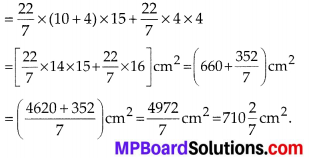Question 4.
A container, opened from the top and made up of a metal sheet, is in the form of a frustum of a cone of height 16 cm with radii of its lower and upper ends as 8 cm and 20 cm, respectively. Find the cost of the milk which can completely fill the container, at the rate of ₹ 20 per litre. Also find the cost of metal sheet used to make the container, if it costs ₹ 8 per 100 cm2. (Take π = 3.14)
Solution:
We have, r1 = 20 cm, r2 = 8 cm and h = 16 cm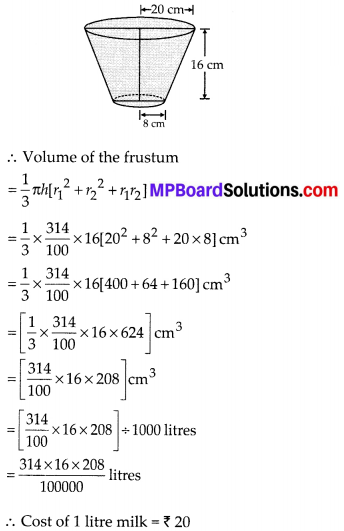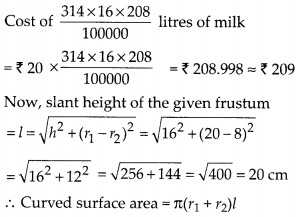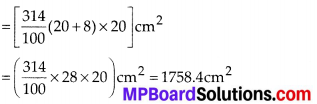Area of the bottom = πr22
= ($$\frac{314}{100}$$ × 8 × 8) cm2 = 200.96 cm2
∴ Total area of metal required
= 1758.4 cm2 + 200.96 cm2 = 1959.36 cm2
Cost of metal required for 100 cm2 = ₹ 8
∴ Cost of metal required for 1959.36 cm2
= ₹ $$\frac{8}{100}$$ × 1959.36 = ₹ 156.75

Question 5.
A metallic right circular cone 20 cm high and whose vertical angle is 60° is cut into two parts at the middle of its height by a plane parallel to its base. If the frustum so obtained be drawn into a wire of diameter $$\frac{1}{16}$$ find the length of the wire
Solution:
Let us consider the frustum DECB of the metallic cone ABC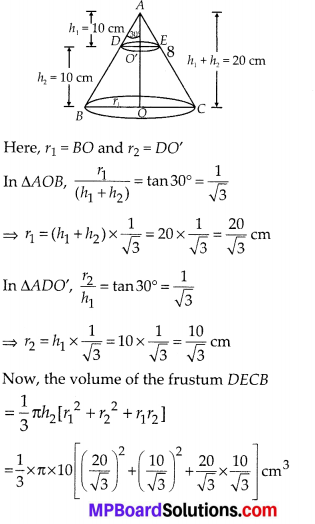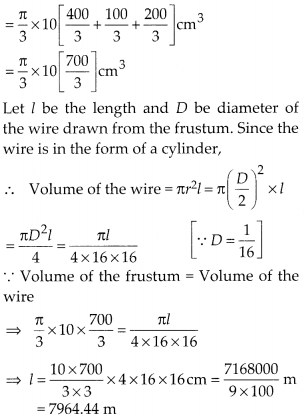Thus, the required length of the wire = 7964.44 m